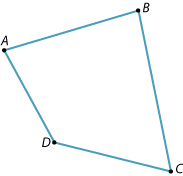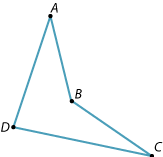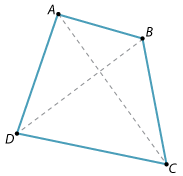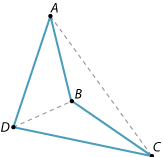A quadrilateral is a plane figure bounded by four straight sides.This quadrilateral is called a convex quadrilateral because none of its four interior angles is a reflex angle.This quadrilateral is called a non-convex quadrilateral because one of its interior angles is a reflex angle.

A quadrilateral has two diagonals that join opposite vertices.